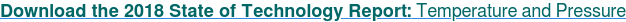Flow / Pressure / Temperature / Level / Weight

# Process control as inventory control

## This concept eases troubleshooting, CV-MV pairing, developing models and more

Often, chemical process control is fundamentally inventory control. Understanding the cause-and-effect of the inventory is key to understanding the process, troubleshooting, choosing appropriate controlled variable-measured variable (CV-MV) pairing, designing cascade or ratio structures, specifying safety overrides and developing models (either first-principles or empirical).

Usually, we don’t measure the inventory. We measure the response to it. Unfortunately, an initial focus on the response often distracts the person from seeing the fundamentals. Recognize the fundamental mechanism. Here are several examples.

## Inventory of gas molecules results in pressure:

A standard air compressor can be an example. The inventory relation of number of molecules to the response can be revealed by the ideal gas law PV = nRT, which leads to:When you release gas or add gas, you're changing the inventory of molecules, and pressure is a response. Note that pressure doesn't flow in or out. Gas molecules are what move, and pressure is the response.

## Inventory of material results in volume, level or weight:

Consider a flow into and out of a right cylindrical tank of height h and area A. Again, the relations are simple. Volume is the product of height and area, and volume times density is mass: V = hA, Vp = m. So, the response, level, is dependent on the inventory of material:To change level, you add or release material. Note that level does not flow in or flow out, but level changes. Material in- and out-flow causes the inventory to change. Level is just a measure of inventory.

The situation is similar for solid accumulation. Consider the integrating process of filling a transport volume with a solids feeder. The weight is the mass flow rate times the duration: W = m ∆t.

Or, more properly, with gravity and dimensional units considered:## Inventory of two materials results in composition:

Consider the continuous blending of two liquids, A and B. Where x represents the mass fraction of component A:Whether the composition is measured by volume fraction, mole fraction, normality, concentration or pH, the measurement is a response of the inventory of A and B, which is related to the inflow of each and the joint outflow.## Inventory of thermal energy (heat) results in temperature:

The relation between heat added and temperature rise is:where ∆T = Tafter - Tbefore heat is added. Then:Again, the controller does not add temperature, it manages processes that add or remove thermal energy, and temperature is the response. It doesn’t matter whether the heater is a flame, or an electrical element, or a chemical or nuclear reaction. It doesn’t matter whether the object is solid, liquid or gas. The analysis is similar. If you want to increase temperature, you don’t add temperature, you add heat. Temperature is the response.

Process control is control of the rates that inventory changes to manage the rates that P, T, V, h, W and X change. Of course, there are exceptions—for example, in flow, we manipulate valve position to increase or decrease flow rate. Here, I don’t see the inventory concept that results in flow rate, so, when we're introduced to control with flow rate examples, it might lead one to miss the inventory concept.

But in most applications, first look for the inventory, then its response, and control the inventory.